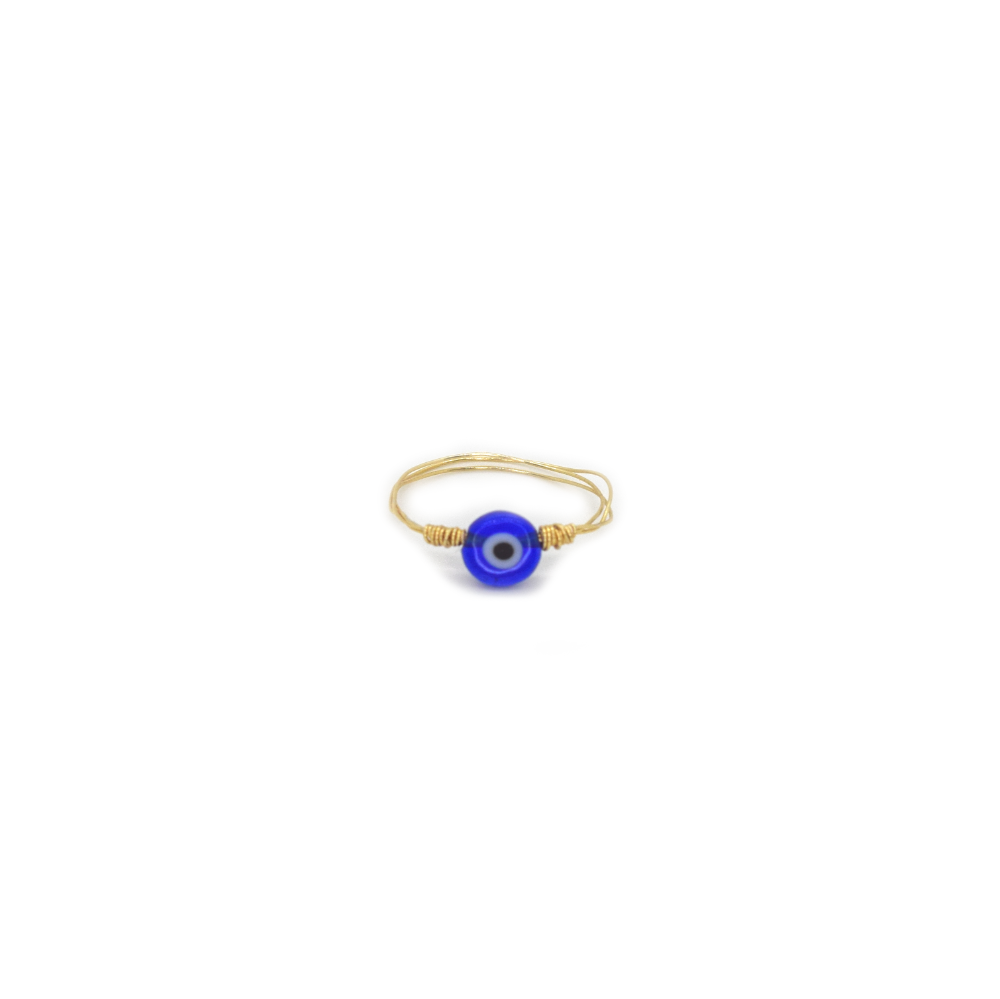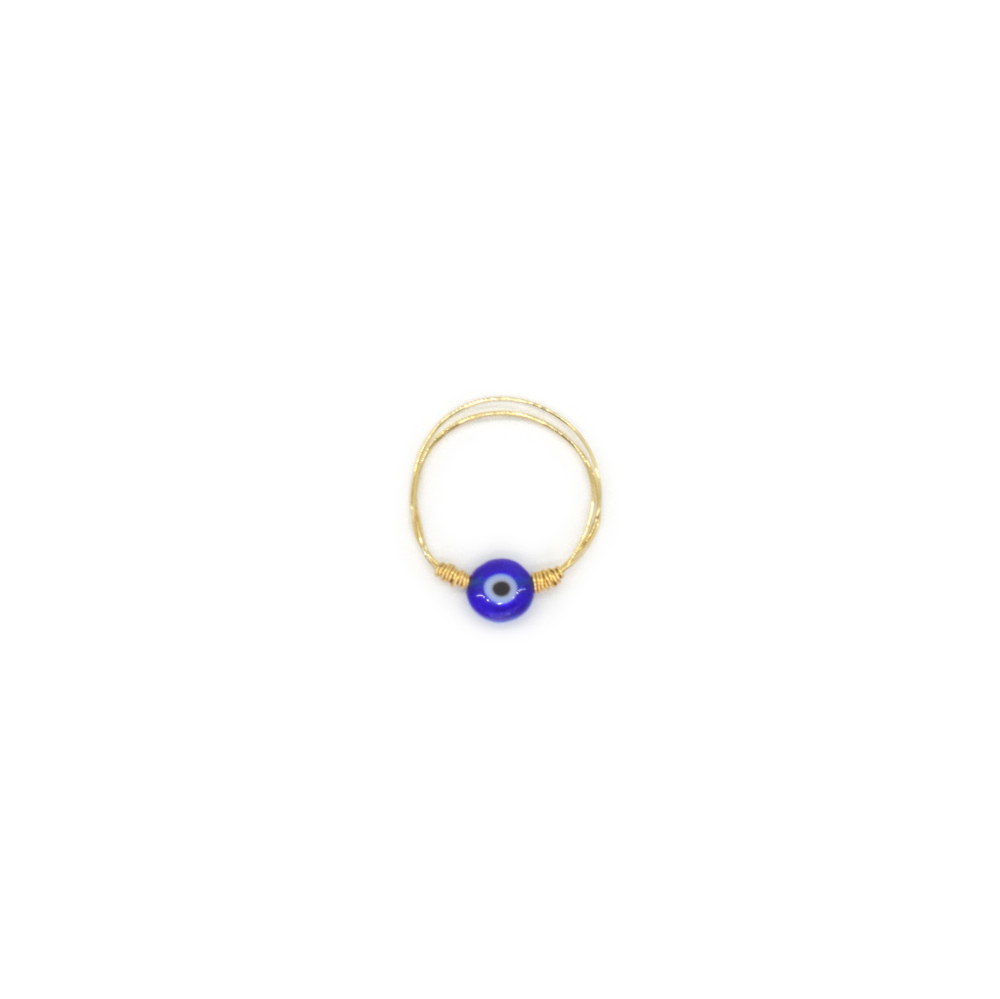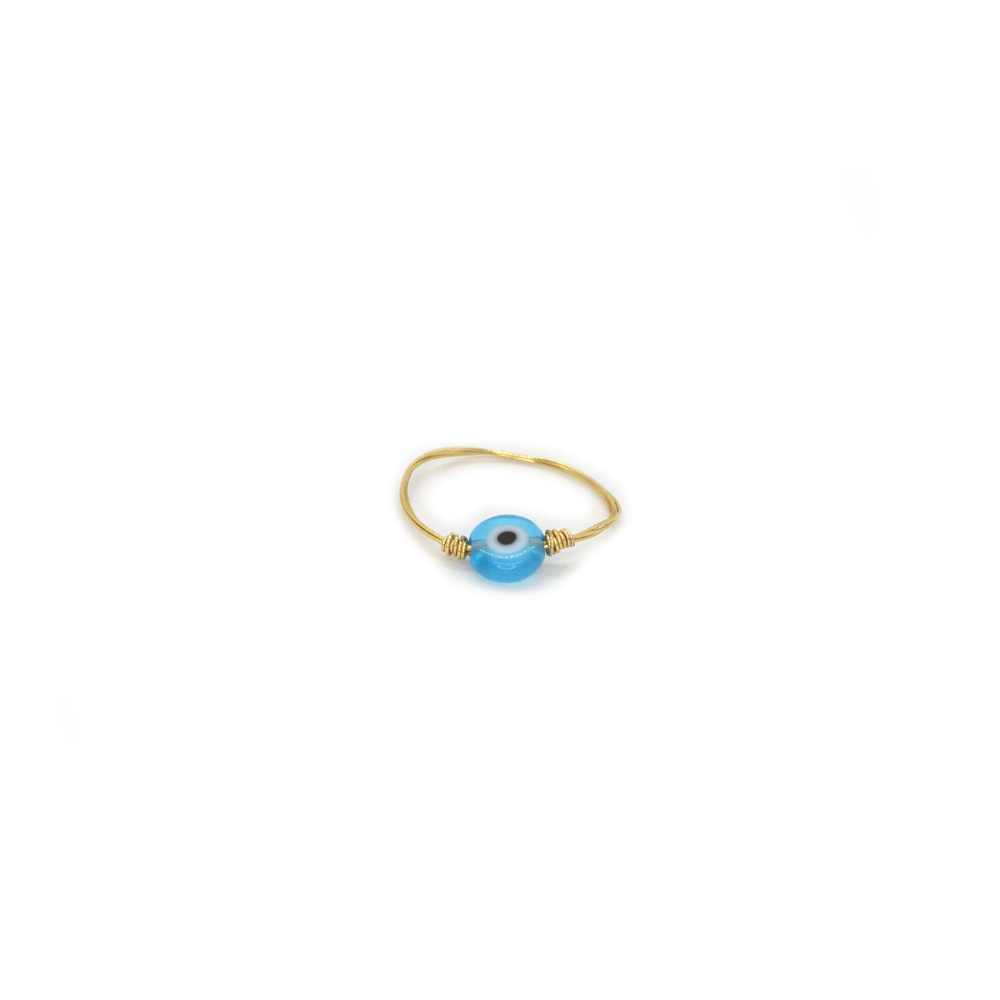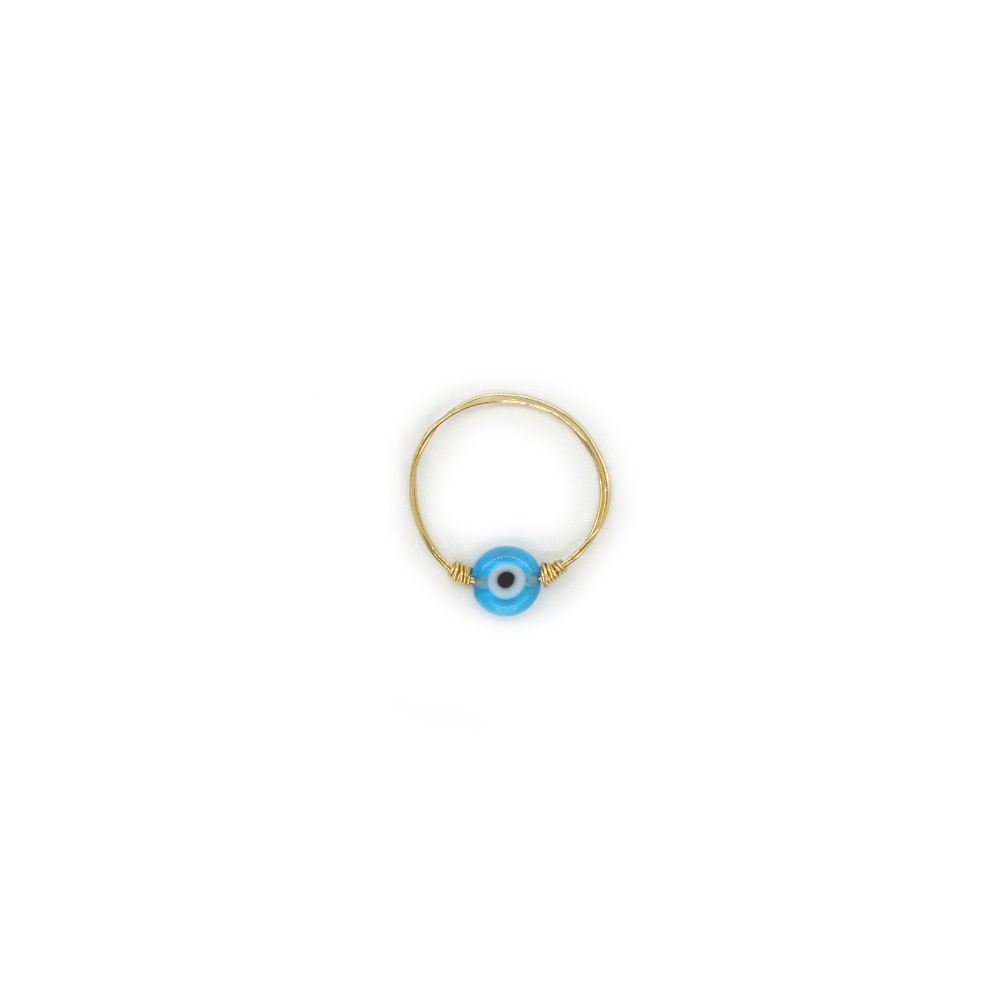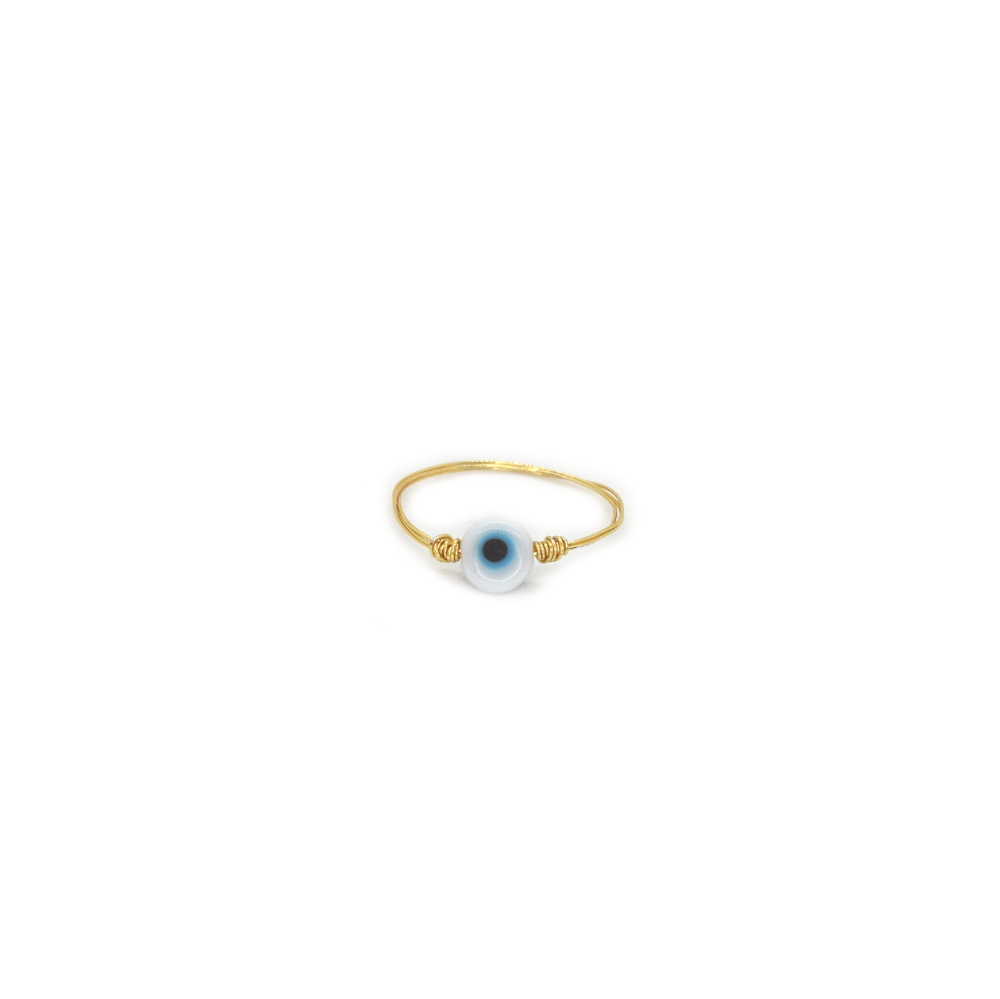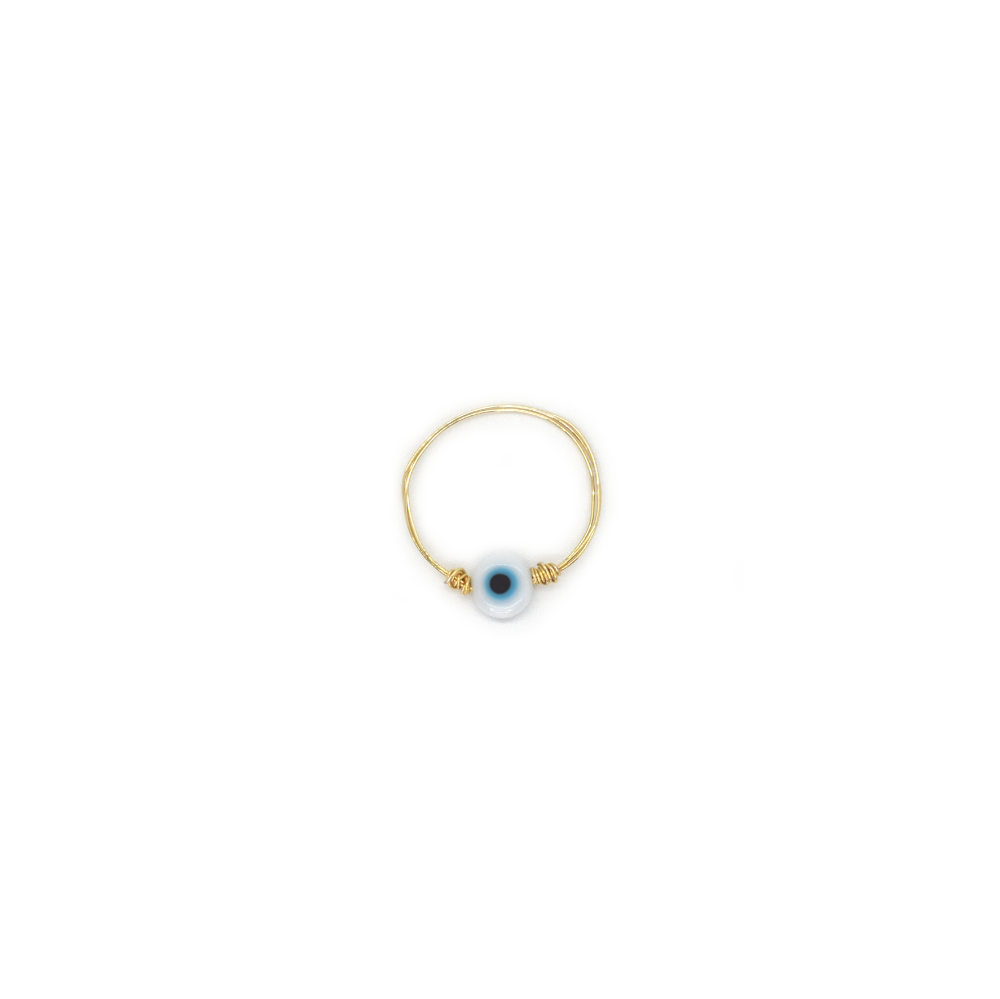On Sale

# Mati POS Ring

Regular price \$30.00

Mati Ring - Gold filled wire, accented with a glass Mati of your choice. Simple clean design to style your wardrobe.

• Fresh Water Pearl Rice Beads
• Ring Sizes measured in mm. ie. (4.25 = 15.1mm
 Ring Sizes 4 = 14.9mm     4.25 = 15.1mm       4.5 = 15.3mm          4.75 = 15.5mm 5 = 15.7mm     5.25 = 15.9mm       5.5 = 16.1mm          5.75 = 16.3mm 6 = 16.5mm     6.25 = 16.7mm       6.5 = 16.9mm          6.75 = 17.1mm 7 = 17.3mm     7.25 = 17.5mm       7.5 = 17.7mm          7.75 = 17.9mm 8 = 18.1mm     8.25 = 18.3mm       8.5 = 18.5mm          8.75 = 18.8mm 9 = 19.0mm     9.25 = 19.2mm       9.5 = 19.4mm          9.75 = 19.6mm 10 = 19.8mm